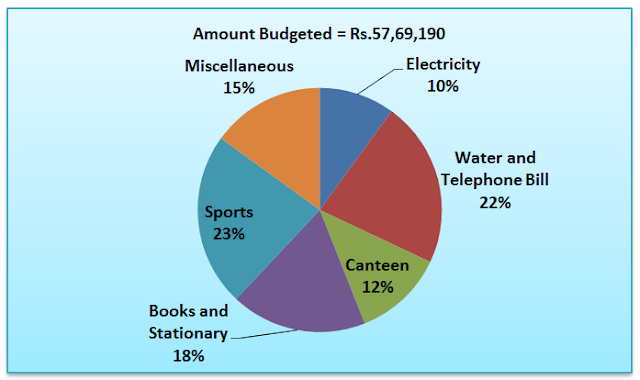# Data Interpretation Practice Set - 5

Data Interpretation Practice Set - 5
Directions (1 – 5): Refer to the following line graph and answer the given questions:
The given line graph and the table shows the data about the income and expenditure of two companies S and T from year 2010 to 2015 in Rs. Crore.Year Income Expenditure 2010 5 : 4 7 : 5 2011 7 : 6 5 : 3 2012 9 : 7 4 : 3 2013 4 : 5 3 : 4 2014 5 : 6 6 : 7 2015 7 : 8 7 : 9
Profit = income-expenditure
Profit%= profit/expenditure*100
1. What is the difference between the profit of company S and T over the year 2010, 2012 and 2014 together in crore?
(a) 21
(b) 14
(c) 25
(d) 17
(e) 18
2. In which year was the income of company T, second lowest?
(a) 2012
(b) 2013
(c) 2010
(d) 2014
(e) Cannot be determined
3. By what percent the profit of company S increased from 2011 to 2012?
(a) 89.9%
(b) 567.1%
(c) 462.5%
(d) 435.1%
(e) None of these
4. The profit percentage of company S in 2015 is approximately what percent of the profit percentage of company T in the same year?
(a) 56%
(b) 123%
(c) 132%
(d) 161%
(e) 171%
5. What is the profit of company T in 2011, 2013 and 2015 together in crore?
(a) 267
(b) 245
(c) 312
(d) 276
(e) None of these
Directions (6 – 10): Refer to the following pie-charts and answer the given questions:
The first pie-chart given below shows the break-up of amount budgeted by a government school for various expenditures in 2013. The second pie chart shows the break-up of funds utilized by the school in 2013.6. What is the approximate ratio of the amount budgeted for Electricity and water and telephone to the funds utilized by Books and stationery, Miscellaneous expenditure and Sports?
(a) 1:1
(b) 1:3
(c) 2:1
(d) 1:1.2
(e) None of these
7. The total expenditure on miscellaneous, books and stationery and sports was closest to the total amount budgeted for?
(a) Sports
(b) Sports and Electricity
(c) Miscellaneous
(d) Canteen
(e) None of these
8. In how many of the given areas the amount budgeted have been fully utilized?
(a) Two
(b) Three
(c) All areas
(d) None
(e) Four
9. 70% of the total expenditure made on Books and stationery was for Books, the amount budgeted for the same was 65% books and stationery. What is the approximate difference between the amount budgeted and the actual expenditure on Books and stationery other than books?
(a) 1,45,231
(b) 1,73,963
(c) 1,56,700
(d) 2,00,002
(e) Cannot be determined
10. If there is an increase of 10% on allocation of funds on sports budgeted, then what percent are funds utilized by sports to new allotted budget for sports?
(a) 45.7%
(b) 46%
(c) 47%
(d) 46.9%
(e) None of these
Solutions:
1. (b), for company S, total profit = (270-224) + (378-288) + (290-252) = 46+90+38= 174 crore
For company T. total profit = (216-160) + (294-216) + (348-294) = 56+78+54 = 188 crore
Therefore required difference = 188-174 = Rs. 14 crore.
2. (b), referring to the table it is clear that in 3013 the income of company T is the second lowest i.e. 267 crore
3. (c), profit gained by company S in 2011 = 336-320 = Rs. 16
Profit gained by company S in 2012 = 378-288 = Rs. 90
Required % = (90-16)/16*100 = 462.5%
4. (e), PROFIT % OF COMPANY s IN 2015 = [(392-287)/287*100] = 36.58%
Profit % of company T in 2015 = [(448-369)/369*100] = 21.4%
Required % = 36.58/21.4 * 100 ≈ 171%
5. (a), profit gained by company T in 2011 = (288-192) = Rs. 96
Profit gained by company T in 2013= (260-168) = Rs. 92
Profit gained by company T in 2015 = (448-369) = Rs. 79
Total profit = 96+92+79 = Rs. 267 crore
6. (a), amount budgeted for Electricity and water & telephone bills = 32/100 * Rs. 57,69,190 ≈ 18, 46,141
Funds utilized by Books and stationery, miscellaneous expenditure and Sports = 55/100* Rs. 3509190 19,89,687
Required ratio = 18, 46,141/1930054 ≈ 1:1
7. (b), expenditure on miscellaneous, books and stationery and sports = 55%* Rs. 35,09,190 ≈ Rs. 19,30,000
Amount budgeted for sports and Electricity = 33% * Rs. 57, 69, 190 ≈ Rs. 19, 40, 000.
After making all combination this is closest to total expenditure on miscellaneous, books and stationery and sports.
8. (d), in the areas where the percentage of funds allocation is greater than or equal to percentage of funds utilization, there funds utilization is definitely more than funds utilization. Such areas are Electricity, water & telephone bill, Canteen and sports.
For books and stationery, funds allocation = 12% of Rs. 57, 69,190≈ Rs. 6, 92,300
Funds utilized = 14% * Rs. 35, 09,190≈Rs. 4, 91,290
For miscellaneous, funds allocation = 15% * Rs. 57, 69, 190≈ Rs. 8, 65, 380
Funds utilized = 18% * Rs. 35, 09,190 = Rs. 6, 31, 650
Thus, for none of the areas the amount budgeted have been fully utilized.
9. (b), Amount budgeted for Books and stationery = 18%* 57, 69,190 ≈10, 38,454,
Amount budgeted for books and stationery other than books = 35% of 10, 38, 454≈3, 63,459.
Total expenditure on Books and stationery = 18% of 35, 09,190 = 6, 31,654.
Total expenditure on books and stationery other than Books = 30% * 6, 31,654 = 1, 89,496.
Required difference = 3, 63,459-1, 89,496 = 1, 73,963.
10. (a), total amount budgeted for sports = 23% * 57,69,190 = 13,26,913.7
10%increase takes place so value = 14, 59,605.07
Now percent = [(19%35,09,190)/ 14,59,605.07] 100 ≈ 45.7%